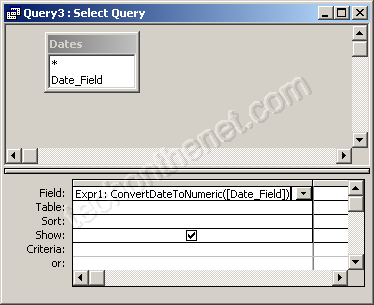## 7/09/2012

### Convert a date to a numeric value

Question: In Access 2003/XP/2000/97, how do you change the date into a numeric value?
For example, I want to change the date 20/02/2004 into the number 20022004. This formats the number as ddmmyyyy.
Answer: In Access, you can create custom functions to supplement what is missing in Access. To do this, click on the Modules tab in the Database window and create a new module. Then paste in the following custom function:

Function ConvertDateToNumeric(pDate As Date) As Long
Dim LYear As Integer
Dim LMth As Integer
Dim LDay As Integer
'Extract the year, month, and day values from the date parameter called pDate
LYear = DatePart("yyyy", pDate)
LMth = DatePart("m", pDate)
LDay = DatePart("d", pDate)
'Format new number as a ddmmyyyy value
ConvertDateToNumeric = Right("00" & CStr(LDay), 2) & Right("00" & CStr(LMth), 2) & CLng(CStr(LYear))
End Function

The function called ConvertDateToNumeric will convert a date into a number using a format of ddmmyyyy.
Next, you'll need to use this function in your query.In the example above, we've used the ConvertDateToNumeric function to convert the field called Date_Field into a number. You will need to substitute your field name with the function. So we've typed ConvertDateToNumeric([Date_Field]) in the first field. Access assigns the field name of "Expr1" - you can always overwrite this.
Now, when we run the query, we'll get the following results: